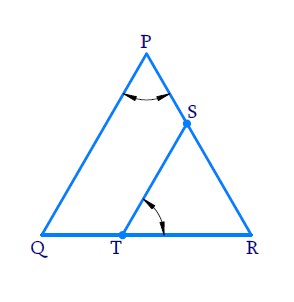# Ex.6.3 Q5 Triangles Solution - NCERT Maths Class 10

Go back to  'Ex.6.3'

## Question

$$S$$ and $$T$$ are points on sides $$PR$$ and $$QR$$ of  $$\Delta PQR$$ such that  $$\angle P{\rm{ }} = \angle RTS$$. Show that

DiagramVideo Solution
Triangles
Ex 6.3 | Question 5

## Text Solution

Reasoning:

If two angles of one triangle are respectively equal to two angles of another triangle, then the two triangles are similar.

This is referred as $$AA$$ criterion for two triangles.

Steps:

In $$\Delta RPQ,\,\,\Delta RTS$$

\begin{align} & \angle RPQ=\angle RTS\,\,\,\,( \text{Given} ) \\ & \angle PRQ=\angle TRS\\&( \text{Common Angle} ) \\\\ & \Rightarrow \Delta RPQ-\Delta RTS\\&( \because \, \text{AA Criterion}) \\ \end{align}

Learn from the best math teachers and top your exams

• Live one on one classroom and doubt clearing
• Practice worksheets in and after class for conceptual clarity
• Personalized curriculum to keep up with school